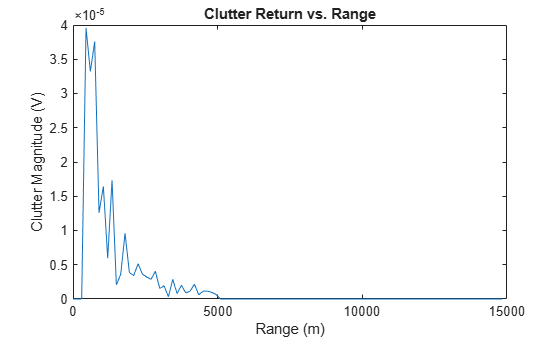# surfacegamma

Gamma value for different terrains

## Syntax

```G = surfacegamma(TerrainType) G = surfacegamma(TerrainType,FREQ) surfacegamma ```

## Description

`G = surfacegamma(TerrainType)` returns the $\gamma$ value for the specified terrain. The $\gamma$ value is for an operating frequency of 10 GHz.

`G = surfacegamma(TerrainType,FREQ)` specifies the operating frequency of the system.

`surfacegamma` displays several terrain types and their corresponding $\gamma$ values. These $\gamma$ values are for an operating frequency of 10 GHz.

## Input Arguments

 `TerrainType` Character vectors that describe the terrain type. Valid values are: `'sea state 3'``'sea state 5'``'woods'``'metropolitan'``'rugged mountain'``'farmland'``'wooded hill'``'flatland'` `FREQ` Operating frequency of radar system in hertz. This value can be a scalar or vector. Default: `10e9`

## Output Arguments

 `G` Value of $\gamma$ in decibels, for constant $\gamma$ clutter model.

## Examples

collapse all

Determine the γ value for a wooded area, and then simulate the clutter return from that area. Assume the radar system uses a single cosine pattern antenna element and has an operating frequency of 300 MHz.

```fc = 300e6; g = surfacegamma('woods',fc); clutter = constantGammaClutter('Gamma',g, ... 'Sensor',phased.CosineAntennaElement, ... 'OperatingFrequency',fc); x = clutter(); r = (0:numel(x)-1)/(2*clutter.SampleRate) * ... clutter.PropagationSpeed; plot(r,abs(x)) xlabel('Range (m)') ylabel('Clutter Magnitude (V)') title('Clutter Return vs. Range')```collapse all

### Gamma

A frequently used model for clutter simulation is the constant gamma model. This model uses a parameter, $\gamma$, to describe clutter characteristics of different types of terrain. Values of $\gamma$ are derived from measurements.

## Algorithms

The $\gamma$ values for the terrain types `'sea state 3'`, `'sea state 5'`, `'woods'`, `'metropolitan'`, and `'rugged mountain'` are from .

The $\gamma$ values for the terrain types `'farmland'`, `'wooded hill'`, and `'flatland'` are from .

Measurements provide values of $\gamma$ for a system operating at 10 GHz. The $\gamma$ value for a system operating at frequency f is:

`$\gamma ={\gamma }_{0}+5\mathrm{log}\left(\frac{f}{{f}_{0}}\right)$`

where ${\gamma }_{0}$ is the value at frequency f0 = 10 GHz.

## References

 Barton, David. “Land Clutter Models for Radar Design and Analysis,” Proceedings of the IEEE. Vol. 73, Number 2, February, 1985, pp. 198–204.

 Long, Maurice W. Radar Reflectivity of Land and Sea, 3rd Ed. Boston: Artech House, 2001.

 Nathanson, Fred E., J. Patrick Reilly, and Marvin N. Cohen. Radar Design Principles, 2nd Ed. Mendham, NJ: SciTech Publishing, 1999.

## Version History

Introduced in R2021a